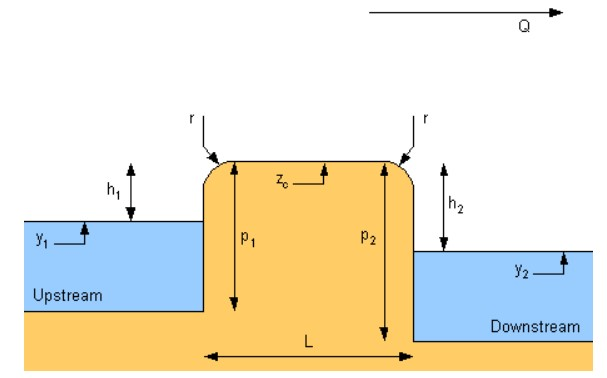Enter the values of head 1,2, and discharge constant along with the width of the weir to calculate flow rate using the broad crested weir calculator.

Formula:
q = Cd × b × h2 × (2g (h1 - h2)) 1/2
m
m
m

Give Us Feedback

The weir flow rate calculator helps to quickly solve the broad crested weir equation by using the inputted values. All the values are required in SI units.

## What is broad crested weir?

Weirs are installed for many purposes on streams and rivers and they have different types.

A broad crested weir is a strong weir made of ceramic or hard material as an obstruction to water or other liquid flow.  It is a flat weir with a net double drop (i.e two head measurements).It is called broadly crested because of its wide edge over the entire stream. But the accuracy of such weirs is obtained through precise calibration.

This weir is usually used over dams in forests and similar places, where the other weirs like V-notch, suffer maintenance problems.

Equations to calculate flow rates are different for different weirs. For broad crested weir, the equation used is:

q = Cd × b × h2 × (2g (h1 - h2)) 1/2

In this equation:

• Cd is discharge coefficient
• b is the breadth of the weir
• h1 and h2 are first and second head drops
• g is the gravitational acceleration

## How to calculate the flow rate of a broad weir?

Example:

For the following set of values for a broad weir, calculate the flow rate.

h1 = 45m

h2 = 42m

Cd = 1

b = 1 m

Solution:

Step 1: Put values in the formula:

q = 1 × 1 × 42 × (2(9.8) (45 - 43)) 1/2

Step 2: Solve:

q = 42 x (2(9.8) (2)) 1/2

q = 42 x 6.261

q = 262.96 m3s-1

### Math Tools